Courses
Courses for Kids
Free study material
Free LIVE classes
More

# How to Count by Ten?## An Introduction to How to Count by 10

Learning to count is fun! It can be done by learning from songs, pictures or any other fun activities. Three stages should be followed to learn this ability most quickly and efficiently as possible. When introducing counting by tens, let us start with the simplest activities.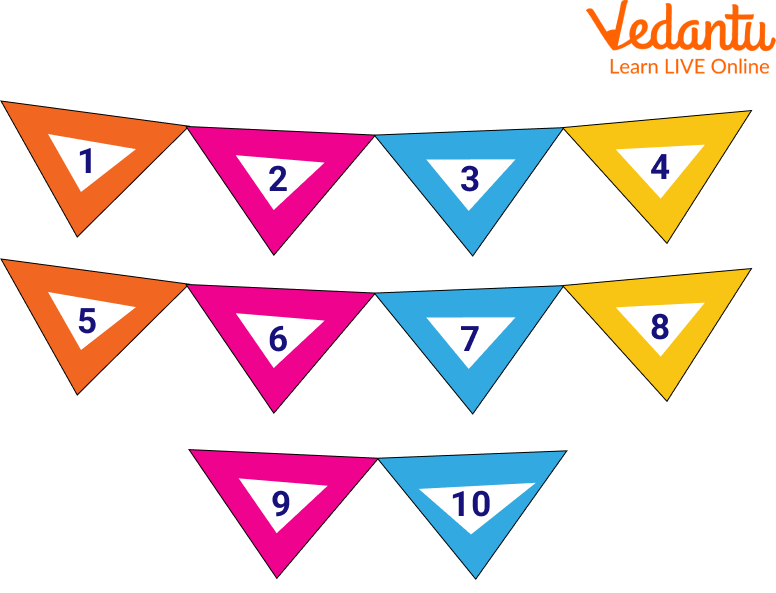Introduction to Counting

After that, move on to activities where we may see and hear the numbers. Start thinking in tens as you enter the third phase. This is assisting kids in connecting their understanding of ten counting to real-world scenarios. It has been discovered that numerous activities are the most successful at imparting this skill.

## Counting by Tens

Because humans have ten fingers, our number system is centred around the number 10.

The ability to think and compute in tens is advantageous for numerous tasks, including estimation, problem-solving, mental addition and subtraction, and many more. Counting in tens is enjoyable! Additionally, kids will have fun playing these games and learn a lot from them. It can be learned from the counting by 10s chart. When we count by tens, we increase a number by tenths of a unit each time.

### 1. Using Numerals To Count By Tens

10, 20, 30, 40, 50, 60, 70, 80, 90

### 2. Counting by Tens and Number Patterns

The numbers form a pattern when you count by tens. Every number has a zero at the end. When you count, the first few digits correspond to the numbers (1, 2, 3, 4, 5, etc.). The digits 10, 20, 30, 40, 50, etc. are produced by this pattern. The numbers in the dark green boxes count by 10.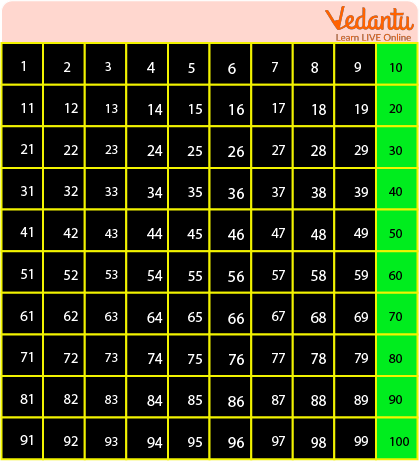Counting by 10

## How to Count by 10?

• Create 10 object groups.

• Start counting out loud still ten, as one, two, three, four, five, six, seven, eight, nine, ten.

• Similarly, we can start the counting as 10, 20, 30, 40, 50, 60, 70, 80, 90, 100.

• It can be learned from the hundred charts also.

The fact that the sum is the same whether you count in 1s or 10s is made tangible by observing these recurring groupings of 10. The counting sequence "10, 20, 30, 40..." can be practised. A hundred chart is another helpful tool for planning and visualising groups of ten as you count by tens.

## Count to 100 by 10s

We can learn it by learning the count to 100 by 10s song which is fun and easy to learn.

The number line shows the series of numbers. Children should count the numbers out loud by seeing the image as 10, 20, 30, 40, 50, 60, 70, 80, 90, 100.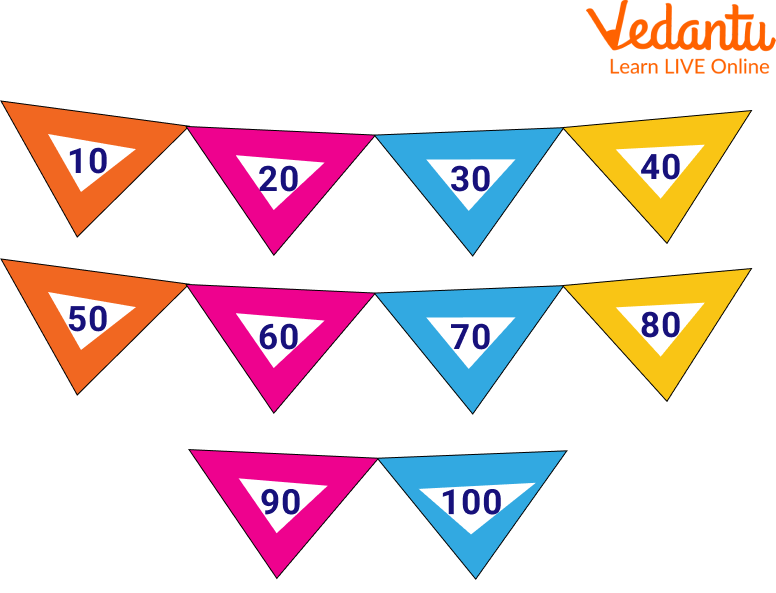Count to 100 by 10s

## Skip Count by 10

Usually, we count as 1,2,3,4,5…. But here we will learn something different. Here, we are going to learn counting by skipping. In this, we skip the 9 steps and straight away jump to the 10th. And we continue the counting as 10, 20, 30, 40, 50, 60, 70, 80, 90, 100.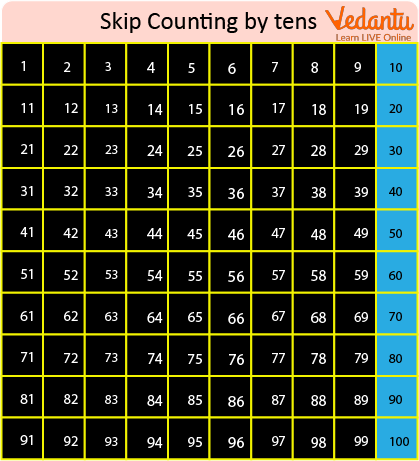Skip Count by 10

## Tens Number Line

Counting by tens can be learned by using the tens number line which is easy to understand. The number line shows the series of numbers. Children should count the numbers out loud by seeing the image as 10, 20, 30, 40, 50, 60, 70, 80, 90, 100.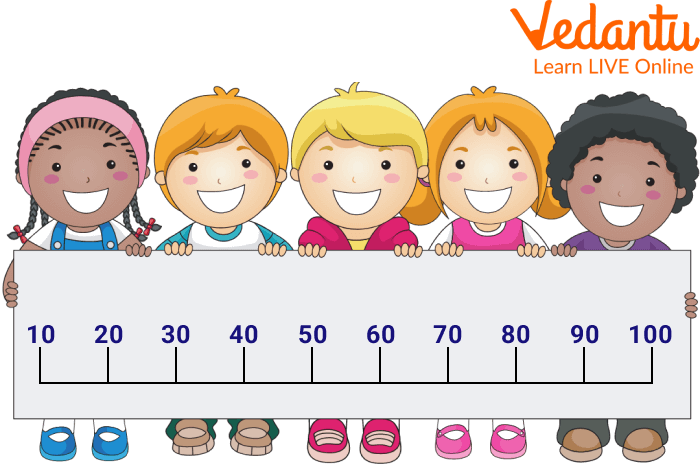Tens Number Line

## Solved Example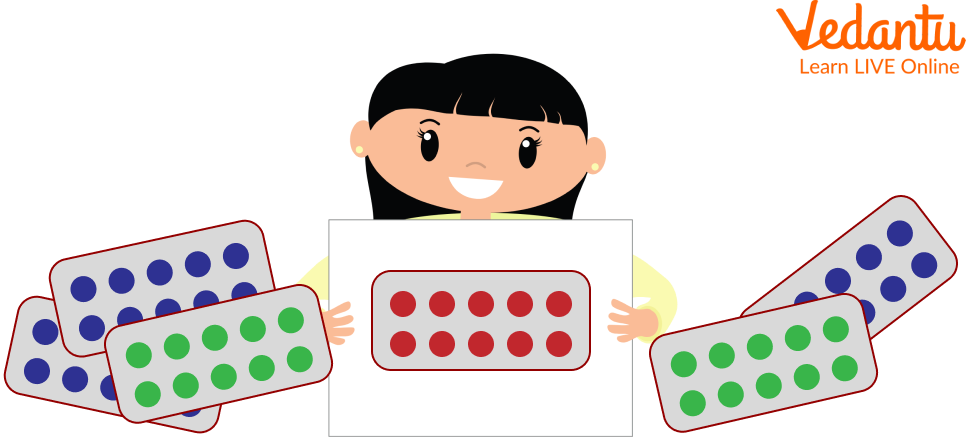The Child likes Collecting Bindis

1. (a) How many packets does she have?

Ans: Six

(b) So how many bindis in all?

Ans: 10 + 10 + 10 + 10 + 10 + 10 = 60

60 bindis in all.

2. Complete the series:

10,20,_,_,_,60,_,_,_,_

Ans: 10, 20, 30, 40, 50, 60, 70, 80, 90, 100

## Practice Questions

1. Complete the skip counting series by 10s:

(i) 20 , 30, ____, ____, ____, ____.

Ans: 20, 30, 40, 50, 60,70

(ii) ____, ____, 40, ____,60.

Ans: 20, 30, 40, 50

## Summary

In this article, we have learned how we can count to 10 easily. We have seen an activity, in which we can learn counting by using either a chart or by reading numerals. Later on, we have seen the concept of skip counting. A skip count of 10s involves either adding 10 to the preceding number or jumping by 10. Every number is 10 more than the one before it. The counting sequence "10, 20, 30, 40..." can be practised to improve fluency when your youngster has realised that counting by tens indicates you're counting several groups of ten more quickly.

Last updated date: 18th Sep 2023
Total views: 92.7k
Views today: 1.92k

## FAQs on How to Count by Ten?

1. How many TENS are there in 60?

Since tens are equal to one group of ten. Here, 6 tens will make 60. Therefore, there are 6 tens in 60.

2. How can one help their kid learn how to use tens and how can you improve at counting in tens?

A game that teaches kids about tens and ones:

Rocks or beans will do. Show that it is simpler to count them in groups of 10 by piling them up on a table. Make ten-group groups first, then separately count the ten groups and the individual beans. One can improve counting in ten by practising the following examples:

• Reading and learning from books.

• A group of children is being counted in a group of 10.

• Utilise a counting stick.

• Chanting Backwards/Forwards.

• The team game Hit The Balloon.

3. What is the use of skip counting?

Kids use skip counting for everyday tasks like telling the time or handling money. It makes calculation faster while counting as we don’t need to count 10 times. Skip counting is essential because it provides a mathematical foundation for children to build on as they learn other mathematical concepts. It also builds up fluency and confidence in them.